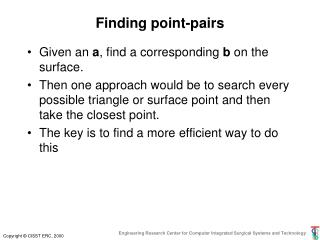DownloadDownload PresentationFinding point-pairs

Finding point-pairs

Download PresentationFinding point-pairs

- - - - - - - - - - - - - - - - - - - - - - - - - - - E N D - - - - - - - - - - - - - - - - - - - - - - - - - - -
Presentation Transcript

1. Finding point-pairs • Given an a, find a corresponding b on the surface. • Then one approach would be to search every possible triangle or surface point and then take the closest point. • The key is to find a more efficient way to do this

2. Find Closest Point from Dense Cloud • Basic approach is to divide space into regions. Suppose that we have one point bk* that is a possible match for a point ak. The distance *=|| bk* - ak|| obviously acts as an upper bound on the distance of the closest point to the surface. • Given a region R containing many possible points bj, if we can compute a lower bound Lon the distance from a to any point in R, then we need only consider points inside R if L < *.

3. Find Closest Point from Dense Cloud • There are many ways to implement this idea • Simply partitioning space into many buckets • Octrees, KD trees, covariance trees, etc.

4. Approaches to closest triangle finding • (Simplest) Construct linear list of triangles and search sequentially for closest triangle to each point. • (Only slightly harder) Construct bounding spheres around each triangle and use these to reduce the number of careful checks required. • (Faster if have lots of points) Construct hierarchical data structure to speed search. • (Better but harder) Rotate each level of the tree to align with data.

5. a r c q p FindClosestPoint(a,[p,q,r]) Hint: For efficiency, work out the least squares problem explicitly. You will have to solve a 2 x 2 linear system for , 

6. E r F D q G A B C p Finding closest point on triangle

7. ProjectOnSegment(c,p,q) p c* q c

8. Bounding Sphere c q b a

9. Bounding Sphere c q b a

10. Hierarchical cellular decompositions

11. Hierarchical cellular decompositions

12. Constructing tree of bounding spheres class BoundingSphere { public: Vec3 Center; // Coordinates of center double Radius; // radius of sphere Thing* Object; // some reference to the thing // bounded };

13. Constructing octree of bounding spheres class BoundingBoxTreeNode { Vec3 Center; // splitting point Vec3 UB; // corners of box Vec3 LB; int HaveSubtrees; int nSpheres; double MaxRadius; // maximum radius of sphere in box BoundingBoxTreeNode* SubTrees; BoundingSphere** Spheres; : : BoundingBoxTreeNode(BoundingSphere** BS, int nS); ConstructSubtrees(); void FindClosestPoint(Vec3 v, double& bound, Vec3& closest); };

14. Constructing octree of bounding spheres BoundingBoxTreeNode(BoundingSphere** BS, int nS) { Spheres = BS; nSpheres = nS; Center = Centroid(Spheres, nSpheres); MaxRadius = FindMaxRadius(Spheres,nSpheres); UB = FindMaxCoordinates(Spheres,nSpheres); LB = FindMinCoordinates(Spheres,nSpheres); ConstructSubtrees(); };

15. Constructing octree of bounding spheres ConstructSubtrees() { if (nSpheres<= minCount || length(UB-LB)<=minDiag) { HaveSubtrees=0; return; }; HaveSubtrees = 1; int nnn,npn,npp,nnp,pnn,ppn,ppp,pnp; // number of spheres in each subtree SplitSort(Center, Spheres, nnn,npn,npp,nnp,pnn,ppn,ppp,pnp); Subtrees = BoundingBoxTree(Spheres,nnn); Subtrees = BoundingBoxTree(Spheres[nnn],npn); Subtrees = BoundingBoxTree(Spheres[nnn+npn],npp); : : }

16. Constructing octree of bounding spheres SplitSort(Vec3 SplittingPoint, BoundingSphere** Spheres, int& nnn, int& npn, … ,int& pnp) { // reorder Spheres(…) into eight buckets according to // comparison of coordinates of Sphere(k)->Center // with coordinates of splitting point. E.g., first bucket has // Sphere(k)->Center.x < SplittingPoint.x // Sphere(k)->Center.y < SplittingPoint.y // Sphere(k)->Center.z < SplittingPoint.z // This can be done “in place” by suitable exchanges. // Set nnn = number of spheres with all coordinates less than // splitting point, etc. }

17. Searching an octree of bounding spheres

18. Searching an octree of bounding spheres void BoundingBoxTreeNode::FindClosestPoint (Vec3 v, double& bound, Vec3& closest) { double dist = bound + MaxRadius; if (v.x > UB.x+dist) return; if (v.y > UB.y+dist) return; …. ; if (v.z < LB.z-dist) return; if (HaveSubtrees) { Subtrees.FindClosestPoint(v,bound,closest); : Subtrees.FindClosestPoint(v,bound,closest); } else for (int i=0;i<nSpheres;I++) UpdateClosest(Spheres[i],v,bound,closest); };

19. Searching an octree of bounding spheres void UpdateClosest(BoundingSphere* S, Vec3 v, double& bound, Vec3& closest) { double dist = v-S->Center;; if (dist - S->Radius > bound) return; Vec3 cp = ClosestPointTo(*S->Object,v); dist = LengthOf(cp-v); if (dist<bound) { bound = dist; closest=cp;}; };